КАТЕГОРИИ:

# Marginal revenue product

The marginal revenue product of labor can also be expressed as:

MRP = MR x MP

where MR (marginal revenue) equals the additional revenue resulting from the sale of an additional unit of output and MP (marginal product, also known as marginal physical product or MPP in many micro principles texts) is the additional output resulting from the use of an additional unit of labor, holding the use of other inputs constant. Suppose, for example, that you wished to compute the marginal revenue product of labor when MR = 4 and MPP = 5. In this case, the employment of an additional worker results in a 5 unit increase in output (holding other inputs constant) while revenue increases by \$4 when an additional unit of output is sold. In this case, the marginal revenue product of labor will equal \$20 (= \$4 x 5).

Using a little bit of algebra, the marginal revenue product can be defined as: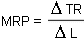Similarly, marginal revenue and marginal product are defined as: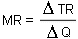and: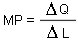The relationship among MRP, MR, and MP can also be seen quite clearly in an algebraic manner: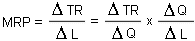Since MRP is equal to the product of MR and MP, to determine the relationship between MRP and the level of labor use, we need to understand how MR and MP change when the level of labor changes.

As you may recall from your micro principles course, the law of diminishing returns can be stated as:

law of diminishing returns - as additional units of a variable input are added to a production process in which other inputs are fixed, the marginal product of the variable input will ultimately decline.

While it is possible that MP may initially increase, a profit-maximizing firm will never hire workers in the range in which this occurs. (To see this, note that if it is profitable to hire the second worker and the third worker has a higher marginal product, it will always be optimal to hire the 3rd worker. A profit-maximizing firm would never hire only two workers in this case.) Thus, marginal product declines over the range of labor use that will be considered by a firm. The diagram below illustrates the relationship between marginal product and the level of labor use.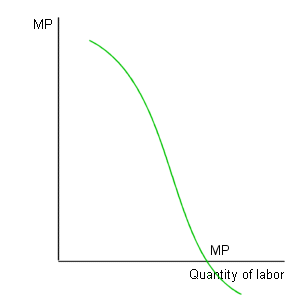As this diagram indicates, it is possible that the marginal product of labor will become negative beyond some level of labor use.

mylektsii.su - Мои Лекции - 2015-2023 год. (0.011 сек.)Все материалы представленные на сайте исключительно с целью ознакомления читателями и не преследуют коммерческих целей или нарушение авторских прав Пожаловаться на материал